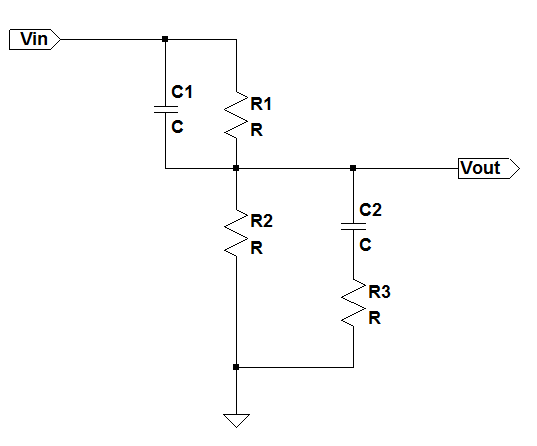# The network [top]

The following circuit shows the lead-lag network which creates 2 poles and 2 zeroes:# Solution [top]

The `\$V_{in}\$` to `\$V_{out}\$` transfer function can be written as:

`\$\$V_{out}/V_{in} = { {(R_3+1/{sC_2})R_2}/{R_3+1/{sC_2}+R_2} }/{ {(R_3+1/{sC_2})R_2}/{R_3+1/{sC_2}+R_2}+R_1/{sR_1C_1+1} } \$\$`

This can be simplified to:
`\$\$V_{out}/V_{in} = { (sR_3C_2+1)R_2(sR_1C_1+1) }/{(sR_3C_2+1)R_2(sR_1C_1+1)+R_1(sC_2(R_2+R_3)+1)}\$\$`

# Zeroes and Poles [top]

So we see the 2 zeros created are:
`\$\$z_1=-1/{R_3C_2}\$\$`

`\$\$z_2=-1/{R_1C_1}\$\$`

The 2 poles can be calculated if we solve the denominator which can we written as:
`\$\$s^2R_1R_2R_3C_1C_2+s(R_1R_2C_1+R_2R_3C_2+R_1C_2(R_2+R_3))+R_1+R_2=0\$\$`

or
`\$\$s^2R_3C_1C_2R_1||R_2+s(R_1||R_2(C_1+C_2)+R_3C_2)+1=0\$\$`

If we substitute the following:
`\$\$A=R_3C_1C_2R_1||R_2\$\$`

`\$\$B=R_1||R_2(C_1+C_2)+R_3C_2\$\$`

we can write (3) as:
`\$\$As^2+Bs+1=0\$\$`

We can solve for s as:
`\$\$s={-B±√{B^2-4A} }/{2A}\$\$`

`\$\$s=-B/{2A}±B/{2A}√{1-{4A}/B^2}\$\$`

Now to simplify further we need to see the relative magnitudes to decide what terms can be neglected. Comparing A and B we can clearly see that if the circuit time constants are lower than even a milli second A ≪ B. That is because A is a product of 2 time constants while B is a time constant. Usually the network is designed to have 1 time constant much smaller than the other. So consider the case when one time constant is 100 times larger than the other. For example `\$R_3C_2 ≫ R_1||R_2C_1\$`. This means that the magnitude of `\$A/B^2\$` = 100/10000 or `\$4A/B^2=4/100\$`. So `\$B^2\$` is still 25 times larger than 4A.

So from this point on the assumption and approximation that we take is:

One time constant in the circuit is more dominant than the other one.

So now we can approximate the poles now as:
`\$\$s≈-B/{2A}±B/{2A}(1-{2A}/B^2)\$\$`

`\$\$p_1≈-1/B ; p_2≈-B/A\$\$`

So substituting the values of A and B in we get the final poles of the circuit as:
`\$\$p_1≈-1/{R_1||R_2(C_1+C_2)+R_3C_2}\$\$`

`\$\$p_2≈-{R_1||R_2(C_1+C_2)+R_3C_2}/{R_3C_1C_2R_1||R_2}\$\$`

If `\$C_1≪C_2\$` and `\$R_3≪R_1||R_2\$` then we can approximate the poles as:
`\$\$p_1≈-1/{R_1||R_2C_2}\$\$`

`\$\$p_2≈-1/{R_3C_1}\$\$`

2. The book Frequency Compensation Techniques for Low-Power Operational Amplifiers (The Springer International Series in Engineering and Computer Science)has good chapter on compensation analysis of multi stage opamps.
3. The book Op Amps for Everyone, Fourth Editionhas a good chapter on different networks for compensating opamps